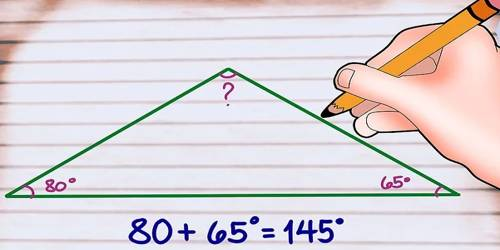Finding the Third Angle of a Triangle

Finding the third angle of a triangle when you know the measurements of the other two angles is easy. All you’ve got to do is subtract the other angle measurements from 180° to get the measurement of the third angle.

The sum of the interior angles of a triangle is equal to 180°. To find the third angle of a triangle when the other two angles are known subtract the number of degrees in the other two angles from 1800.

Example: How many degrees are in the third angle of a triangle whose other two angles are 40° and 650? Answer: 1800 – 400 – 650 = 750

When two angles are known but the third is unknown, we can calculate the unknown angle. To find the third unknown angle of a triangle, subtract the number of degrees in the other two angles from 180°.

Example: How many degrees are in the third angle of a triangle whose other two angles are 40° and 65°?

Answer: 180° – 40° – 65° = 75°

Add up the two known angle measurements. All you have to know is that all of the angles in a triangle always add up to 180°. This is true 100% of the time. So, if you know two of the three measurements of the triangle, then you’re only missing one piece of the puzzle. The first thing you can do is add up the angle measurements you know. In this example, the two angle measurements you know are 80° and 65°. Add them up (80° + 65°) to get 145°.Subtract this number from 180°. The angles in a triangle add up to 180°. Therefore, the remaining angle must make the sum up the angles up to 180°. In this example, 180° – 145° = 35°.

Write down your answer. You now know that the third angle measures 35°. If you’re doubting yourself, just check your work. The three angles should add up to 180° for the triangle to exist. 80° + 65° + 35° = 180°. You’re all done.

Conclusion

The key to finding the missing angle measure of a triangle is to remember that the sum of the interior angles of any triangle is always 180°. If you know 2 angles then you can subtract their sum from 180° to find the measure of the 3rd angle.

Information Source;